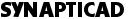Return to: A Translation Example page ```**********************************--- -- VHDL File of a JTAG Tap Controller **********************************--- library ieee; use ieee.std_logic_1164.all; entity tap_controller is port (tms : in std_ulogic; tck : in std_ulogic; trst : in std_ulogic; sel : out std_ulogic; enable : out std_ulogic; test_logic_reset : out std_ulogic; idle : out std_ulogic; resetn : out std_ulogic; shift_ir : out std_ulogic; update_ir : out std_ulogic; clock_ir : out std_ulogic; shift_dr : out std_ulogic; capture_dr : out std_ulogic; clock_dr : out std_ulogic; update_dr : out std_ulogic); end tap_controller; library mgc_bscan; architecture BEHAVIORAL of tap_controller is signal D : std_ulogic := '1'; signal C : std_ulogic := '1'; signal B : std_ulogic := '1'; signal A : std_ulogic := '1'; signal NA : std_ulogic; signal NB : std_ulogic; signal NC : std_ulogic; signal ND : std_ulogic; signal GRST : std_ulogic; signal GENB : std_ulogic; signal GSIR : std_ulogic; signal GSDR : std_ulogic; signal tckd : std_ulogic; signal trsthi : std_ulogic; signal tmsbuf : std_ulogic; -- buffered signal trstnbuf : std_ulogic; -- buffered -- concurrent statements to define output logic. begin trsthi <= not trstnbuf; SEL <= "D;" tckd <= "not" tck; CLOCK_IR <= "not" (not A and B and D and tckd); UPDATE_IR <= "A" and not B and C and D and tckd; CLOCK_DR <= "not" (not A and B and not D and tckd); test_logic_reset <= "A" and B and C and D; test-logic-reset idle <= "not" A and not B and C and D; run-test/idle UPDATE_DR <= "A" and not B and C and not D and tckd; CAPTURE_DR <= "not" A and B and C and not D; tmsbuf <= "tms;" trstnbuf <= "trst;" -- concurrent statements to define next state logic. NA <= "not((not(not" tmsbuf and (not C and A)) and not(tmsbuf and not B)) and (not(tmsbuf and not A) and not(tmsbuf and (C and D)))); NB <= "not((((((not(not" tmsbuf and (B and not A)) and not(not tmsbuf and not C)) and not(not tmsbuf and (not D and B))) and not(not tmsbuf and (not D and not A))) and not(tmsbuf and (C and not B))) and not((tmsbuf and D) and (C and A)))); NC <= "not(not(C" and not B) and (not(C and A) and not(tmsbuf and not B))); ND <= "not((not(D" and not C) and not(D and B)) and (not(not tmsbuf and (C and not B)) and not((not D and C) and (not B and not A)))); ---Combinational logic GRST <= "not((A" and B) and (C and D)); GSIR <= "(not" A and B) and (not C and D); -- made active high by mrd GSDR <= "(not" A and B) and (not C and not D); GENB <= "not(not" GSIR and not GSDR); GENB <= "GSIR" or GSDR; -- Define D inputs and sets to four state FFs process (TRSTHI, TCKD) begin if TRSTHI="1" then RESETN <= "0" ; elsif rising_edge(TCKD) then RESETN <= "GRST;" end if; end process; process (TRSTHI, TCKD) begin if TRSTHI="1" then ENABLE <= "0" ; elsif rising_edge(TCKD) then ENABLE <= "GENB;" end if; end process; process (TRSTHI, TCKD) begin if TRSTHI="1" then SHIFT_IR <= "1" ; elsif rising_edge(TCKD) then SHIFT_IR <= "GSIR;" end if; end process; process (TRSTHI, TCKD) begin if TRSTHI="1" then SHIFT_DR <= "1" ; elsif rising_edge(TCKD) then SHIFT_DR <= "GSDR;" end if; end process; -- Define D inputs and resets to four output FFs. process (TRSTHI, tck) begin if trsthi="1" then D <= "1" ; elsif rising_edge(tck) then D <= "ND;" end if; end process; process (trsthi, tck) begin if trsthi="1" then C <= "1" ; elsif rising_edge(tck) then C <= "NC;" end if; end process; process (trsthi, tck) begin if trsthi="1" then B <= "1" ; elsif rising_edge(tck) then B <= "NB;" end if; end process; process (trsthi, tck) begin if trsthi="1" then A <= "1" ; elsif rising_edge(tck) then A <= "NA;" end if; end process; end BEHAVIORAL; ``` Back to VHDL2Verilog Index Phone: 540-953-3390 | Email: Sales OfficeCOPYRIGHT 2019, SYNAPTICAD SALES, INC., ALL RIGHTS RESERVED.